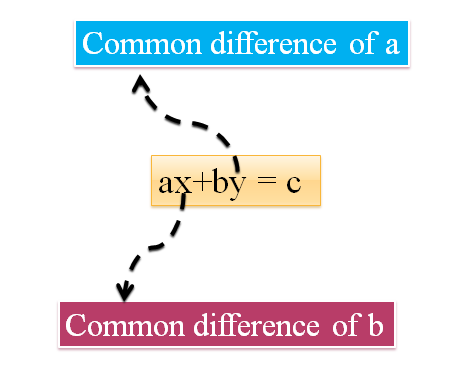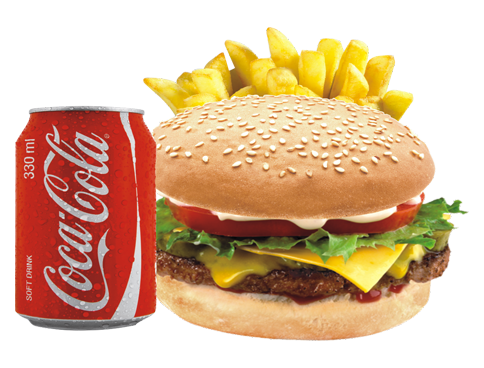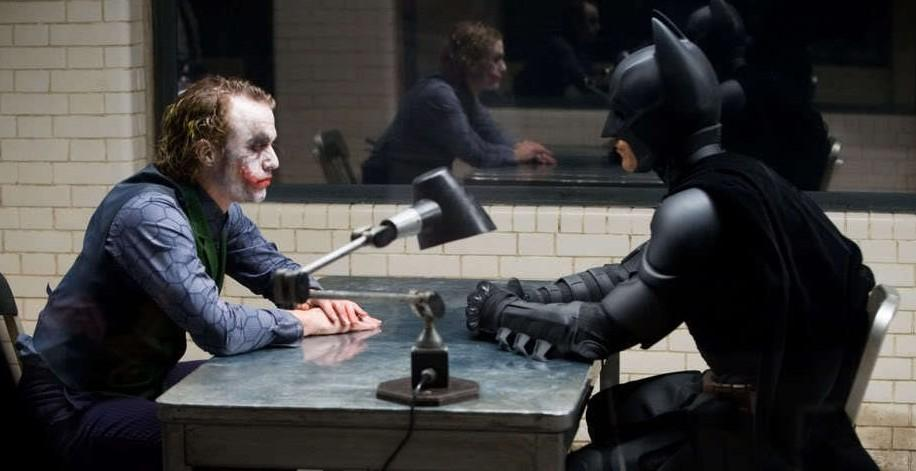# Algebra

#### FUNCTIONS-1

Linear Equations: 2

Special Equations:

Special Equations are an extension of linear equations in two variables; where there is only one equation given and based on that one has to find the solutions satisfying the conditions. There can be exactly one solution or even more than that and the question might even ask the possible number of solutions for the same.

Let’s take an example:

Let us say we have an equation   : x + 3y = 90

Now, this equation will have infinite number of real solutions. We can put a constraint to find number of non negative integral solutions for this equation.

The different solutions satisfying the equation are (0,30), (3,29),(6,28),……(90,0)

Now an interesting pattern follows here- the values of x satisfying the above equation are in A.P., with the common difference of 3, while the values of y are also in an Arithmetic Progression with a common difference of 1. The values of x are increasing from 0 to 90, while the values of y are decreasing from 30 to 0. Thus, there are in all 31 non negative solutions.

If we were asked the number of natural number solutions? The answer would be 29 as we will remove the two solutions-(0,30) and (90,0).

In general we can say that, if we have an equation ax +by = c, then the values of x will be at a common difference of ‘b’ while the values of y will be at a common difference of ‘a’. Also if we are increasing the values of x, then the values of y will decrease and vice versa.Now let us say we have an equation : x-3y = 90 and we are interested to find the non negative integral solutions of this equation. The possible solutions are (90,0),  (93,1), (96,2),……

Here again the values of x are at a common difference of 3 and the values of y are at the common difference of 1. The only difference being if x is increasing, the y will also increase and vice versa.

Example1: Find the number of non negative integral solution for 2x+y = 100.

Solution: 2x +y =100.

The values of x will be at the common difference of 1(coefficient of y) and the values of y will be at the common difference of 2 (coefficient of x)

The first pair will be (50,0) now the other solutions become (49,2), (48,4)…..(0,100)

So, there are 51 non integral solutions to the above equation.

Example 2: Find the number of natural number solutions to the equation 4x +6y = 400

Solution: The equation is 4x+6y = 400.

Now here the import ant point to note is that the coefficients of x and y are not co-prime. So, first we’ll reduce them to relatively prime numbers and then solve it.

Dividing the entire equation by 2, we get: 2x +3y = 200

Now, the values of x will be at a common difference of 3 and the values of y will be at a common difference of 2. Also as x increases, y will decrease and vice versa.

The solutions are (100,0), (97,2), (94,4),……..(1,66)

Here the values of y start from 0 to 66 with a common difference of 2. So, there are 34 non integral solutions.

But as we have to find only the natural solutions, so subtracting the pair (100,0) from the list, we get 33 natural number solutions.

Example 3: Find the number of natural number solutions for the equation

7x +12y =  215

Solution: The equation is 7x +12y = 215.

So now, the values of x will be at a common difference of 12 and the values of y will be at a common difference of 7. Also as x increases, y will decrease and vice versa.

But the problem is to find the first solution of this equation and then we can apply the concept of A.P.

7x + 12y = 215

7x = 215 -12y

As the L.H.S. is a multiple of 7, breaking R.H.S. into multiples of 7 we get

7x = 210 + 5 -7y – 5y

7x = 210 -7y + 5(1-y)

For R.H.S. to be a multiple of 7, 5(1-y) must also be a multiple of 7, so for y = 8, we get it a multiple of 7.

Thus, x = 17 and y = 8 is the first solution.

The other solution will be (5,15) and we’ll stop here as we need only natural number solutions.

So (17,8) and (5,15) are the required natural number solutions.

Example 4: Solve for integral values: 8x – 11y = 325  for -25 ≤ y ≤ 25

Solution : The given equation is 8x -11y =325

The values of x will be at a common difference of 11 while that of y will be at the common difference of 8. Also, if x increases y will also increase (as the difference is constant) and vice versa.

8x = 325 + 11y

L.H.S. is a multiple of 8, so breaking the R.H.S. into multiples of 8 we get,

8x = 320 + 8y + 5 +3y

So, 5 +3y should be a multiple of 8 giving one value of y as 1.

So x = 42 and y = 1

(42,1) is a solution. Also we need to check values for which -25 ≤ y ≤ 25

(9,-23),(20,-15)  (31,-7) (42,1), (53,9), (64,17), (75,25) are the possible solutions.

Thus, there are 7 solutions in the given range.

Example 5: If the cost of one pen is Rs 17 while that of one eraser is Rs 13, then how many pens and erasers can a person buy for Rs 279?

Solution: Let the number of pens be ‘x’ and the number of eraser be ‘y’

According to the condition: 17x + 13y = 279

13y = 279 -17x

As L.H.S. is a multiple of 13, breaking the R.H.S. into a multipe of 13 we get

13y = 273 -13x + 6 -4x = 273 -13x +2(3-2x)

So, 3-2x must be a multiple of 13 giving x = 8 and y = 11.

This is the only solution here.

So, number of pens is 8 and number of erasers that can be bought is 11.

Example 6:Source: burger perfect

A bottle of coke costs Rs 9 while a burger costs Rs 20. If Hanu has an amount of Rs 576, then how many solutions exist for the number of  bottles of coke and burgers can he buy if it is given that the total of bottles and burgers bought is a prime number?

Solution: Let the number of bottles of coke be ‘x’ and number of burgers be ‘y’.

According to the condition: 9x + 20y = 576

9x = 576 – 20y

As the L.H.S. is a multiple of 9, breaking the R.H.S. into multiples of 9 we get:

9x = 576 -18y -2y

So 2y must be a multiple of 9 or y is a multiple of 9

The least value of y = 0 and so x = 64

Thus, the solutions satisfying the condition will be (64,0),(44,9),(24,18),(4,27)

Thus, 4 solutions are possible out of which only for (44,9) and (4,27) that we get the total to be a prime number.

Example 7:Source: tufts

Joker challenged the Batman to appear in a test in which there were 100 questions. The correct answer would give 4 marks while a wrong answer would deduct 1 mark, the unanswered questions would fetch the Batman no marks. In how many ways can the Batman score a total of 70 marks in the test such that the number of unattempted questions is not less than 50% of the total number of questions in the paper?

Solution: Let the number of correct answers, wrong answers and un-attempted questions be C,W and U respectively.

C + W + U =100

Also 4C –W = 70

4C = 70 + W

4C = 68 + 2 + W

Minimum value of W = 2 and so C = 18

The table below gives the solutions along with the number of unanswered questions.

 Correct Wrong Un-attempted Total 18 2 80 100 19 6 75 100 20 10 70 100 21 14 65 100 22 18 60 100 23 22 55 100 24 26 50 100

Thus there are 7 ways in which the Batman can score 70 marks without letting the un- answered questions to be less than 50% of the total number of questions.